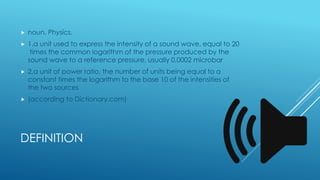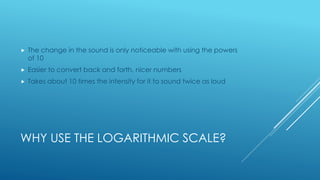Diese Präsentation wurde erfolgreich gemeldet.×

1 von 6
1 von 6

# Decibels

Decibels scale

Decibels scale

### Decibels

1. 1. DECIBELS
2. 2. DEFINITION  noun, Physics.  1.a unit used to express the intensity of a sound wave, equal to 20 times the common logarithm of the pressure produced by the sound wave to a reference pressure, usually 0.0002 microbar  2.a unit of power ratio, the number of units being equal to a constant times the logarithm to the base 10 of the intensities of the two sources  (according to Dictionary.com)
3. 3. WHY USE THE LOGARITHMIC SCALE?  The change in the sound is only noticeable with using the powers of 10  Easier to convert back and forth, nicer numbers  Takes about 10 times the intensity for it to sound twice as loud
4. 4. DIFFERENCE IN DECIBELS  Difference in decibels used 10𝑙𝑜𝑔 𝑃2 𝑃1 𝑑𝐵 where log = base 10  Can see that inverse power ratios will create the opposite sign of the same number of decibels  Eg. 10 log 1 2 = −3 𝑑𝐵 10 log 1 = 0 𝑑𝐵 10 log 2 = 3 𝑑𝐵 10 log 10 = 10 𝑑𝐵 http://www.animations.physics.unsw.edu.au/jw/dB.htm
5. 5. SCALE  Important to protect your ears  Do not spend more than 30 minutes at 110 dB because the damage can be permanent  Use ear plugs if needed
6. 6. REFERENCES  http://www.animations.physics.unsw.edu.au/jw/dB.htm  http://www.gcaudio.com/resources/howtos/loudness.html